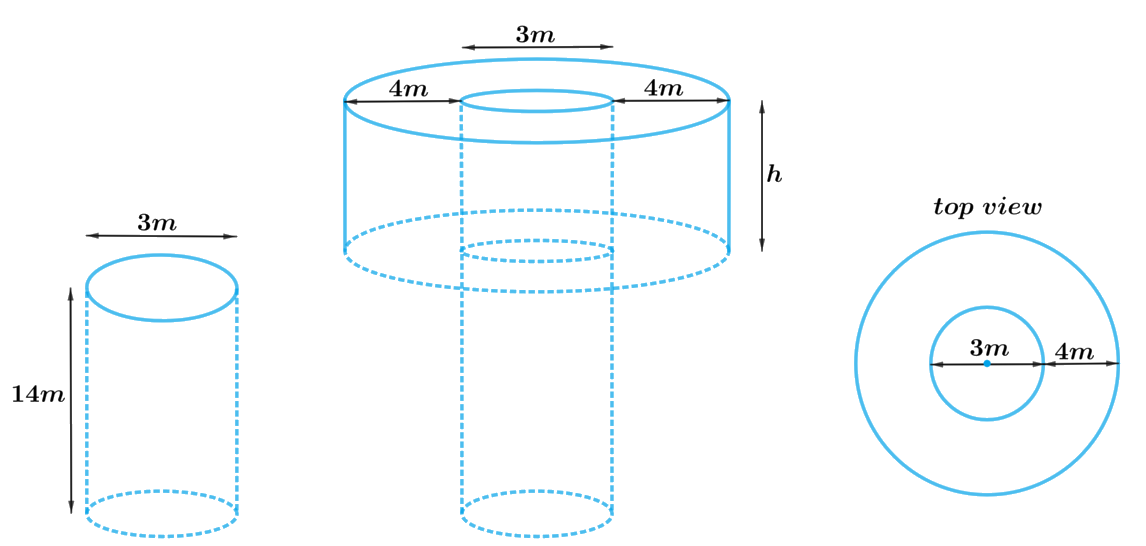# Ex.13.3 Q4 Surface Areas and Volumes Solution - NCERT Maths Class 10

Go back to  'Ex.13.3'

## Question

A well of diameter $$3\,\rm{m}$$ is dug $$14\,\rm{m}$$ deep. The earth taken out of it has been spread evenly all around it in the shape of a circular ring of width $$4\,\rm{m}$$ to form an embankment. Find the height of the embankment.

Video Solution
Surface Areas And Volumes
Ex 13.3 | Question 4

## Text Solution

What is known?

Depth of the well is $$14\rm{m}$$ and diameter is $$3\rm{m}$$. Width of the circular ring of the embankment is $$4\rm{m}$$.

What is unknown?

The height of the embankment.

Reasoning:

Draw a figure to visualize the shapes betterFrom the figure, it can be observed that the shape of the well will be cylindrical, and earth evenly spread out to form an embankment around the well in a circular ring will be in a cylindrical shape (Hollow cylinder) having outer and inner radius.

Volume of the earth taken out from well $$=$$ Volume of the earth used to form embankment

Hence, Volume of the cylindrical well $$=$$ Volume of the hollow cylindrical embankment

We will find the volume of the hollow cylindrical embankment by subtracting volume of inner cylinder from volume of the outer cylinder.

Volume of the cylinder $$= \pi {r^2}h$$

where $$r$$ and $$h$$ are the radius and height of the cylinder respectively.

Steps:

Depth of the cylindrical well,$${h_1} = 14 \rm m$$

Radius of the cylindrical well,\begin{align}r = \frac{3}{2} \rm m = 1.5m\end{align}

Width of embankment $$= 20 \rm m$$

Inner radius of the embankment,\begin{align}r = \frac{3}{2} \rm m\end{align}

Outer radius of the embankment, R $$=$$ Inner radius $$+$$ Width

\begin{align}R &= 1.5 \rm m + 4m\\&= 5.5 \rm m\end{align}

Let the height of embankment be $$h$$

Volume of the cylindrical well $$=$$ Volume of the hollow cylindrical embankment

\begin{align}\pi {r^2}{h_1} &= \pi {R^2}h - \pi {r^2}h\\\pi {r^2}{h_1} &= \pi h\left( {{R^2} - {r^2}} \right)\\{r^2}{h_1} &= h\left( {R - r} \right)\left( {R + r} \right)\\h &= \frac{{{r^2}{h_1}}}{{\left( {R - r} \right)\left( {R + r} \right)}}\\&= \frac{{{{\left( {1.5 \rm m} \right)}^2} \times 14 \rm m}}{{ \begin{bmatrix}\left( {5.5\rm m - 1.5m} \right) \\ \left( {5.5 \rm m + 1.5} \right) \end{bmatrix}}}\\&= \frac{{2.25\rm {m^2} \times 14m}}{{4 \rm m \times 7m}}\\&= 1.125 \rm m\end{align}

Therefore, the height of the embankment will be $$1.125\rm{ m.}$$

Learn from the best math teachers and top your exams

• Live one on one classroom and doubt clearing
• Practice worksheets in and after class for conceptual clarity
• Personalized curriculum to keep up with school